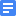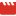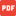Calculating Conservation of Energy Notes TemplateCalculating Conservation of Energy.mp4Conservation of Energy Notes TemplateConservation of Energy Practice KEY.pdfConservation of Energy ProblemsKinetic and Potential Energy Notes TemplateKinetic and Potential Energy Practice KEY.pdfKinetic and Potential Energy ProblemsKinetic Potential Energy.mp4Law of Conservation of Energy.mp4Power Notes TemplatePower.mp4Stair Climbing and Power.pdfUnit 4 WorkPowerEnergy FLIPPED.pdfWork and Power Practice KEY.pdfWork and Power ProblemsWork Notes TemplateWork-Energy Theorem Notes TemplateWork-Energy Theorem.mp4